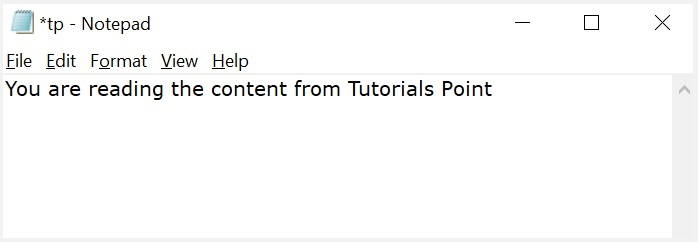# How to read and write a file using Javascript?

The read and write operations in a file can be done by using some commands. But the module which is required to perform these operations is to be imported. The required module is ‘fs’ which is called as File System module in JavaScript.

## Write operation on a file

After the File System file is imported then, the writeFile() operation is called. The writeFile() method is used to write into the file in JavaScript. The syntax of this method is as follows −

writeFile(path,inputData,callBackFunction)


The writeFile() function accepts three parameters −

• Path − The first parameter is the path of the file or the name of the file into which the input data is to be written.

If there is a file already, then the contents in the file are deleted and the input which is given by the user will get updated or if the file is not present, then the file with that will be created in the given path and the input information is written into it.

• inputData − The second parameter is the input data which contains the data to be written in the file that is opened.

• callBackFuntion − The third parameter is the function which is the call back function which takes the error as the parameter and shows the fault if the write operation fails.

### Example 1

Following is an example of the write operation in files in JavaScript.

const fs = require('fs')
let fInput = "You are reading the content from Tutorials Point"
fs.writeFile('tp.txt', fInput, (err) => {
if (err) throw err;
else{
console.log("The file is updated with the given data")
}
})


If you open input file you can observe the written data in it as shown below −After the File System module is imported, the reading of the file in JavaScript can be done by using the readFile() function.

### Syntax

The syntax to read from a file is as follows −

readFile(path, format, callBackFunc)


The readFile() function accepts three parameters including one optional parameter.

• Path − The first parameter is the path of the test file from which the contents are to read. If the current location or directory is the same directory where the file which is to be opened and read is located then, only the file name has to be given.

• Format − The second parameter is the optional parameter which is the format of the text file. The format can be ASCII, utf-8 etc.

• CallBackFunc − The third parameter is the call back function which takes the error as the parameter and displays the fault is any raised due to the error.

### Example 2

Following example tries to read the contents of the file populate in the previous example and print it −

const fs = require('fs')
if (err) throw err;
console.log(inputD.toString());
})


### Output

Following is the output of the above example −

You are reading the content from Tutorials Point


The text which is displayed in the console is the text which is in the given file.

### Example 3

Following is a combined example of the above of reading and writing files using the fs module on node.js. Let us create a JS file named main.js having the following code −

var fs = require("fs");
console.log("Going to write into existing file");

// Open a new file with name input.txt and write Simply Easy Learning! to it.
fs.writeFile('input.txt', 'Simply Easy Learning!', function(err) {
console.log("Data written successfully!");